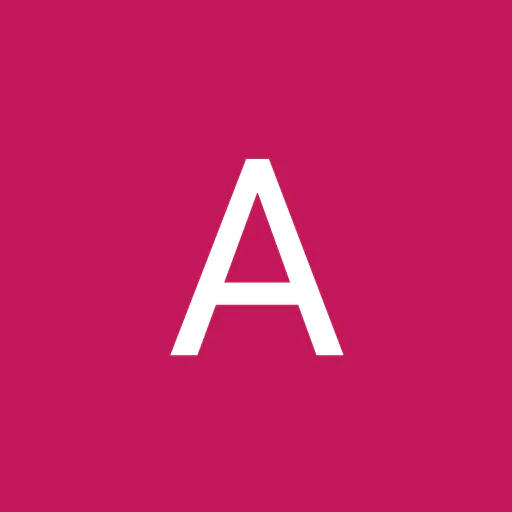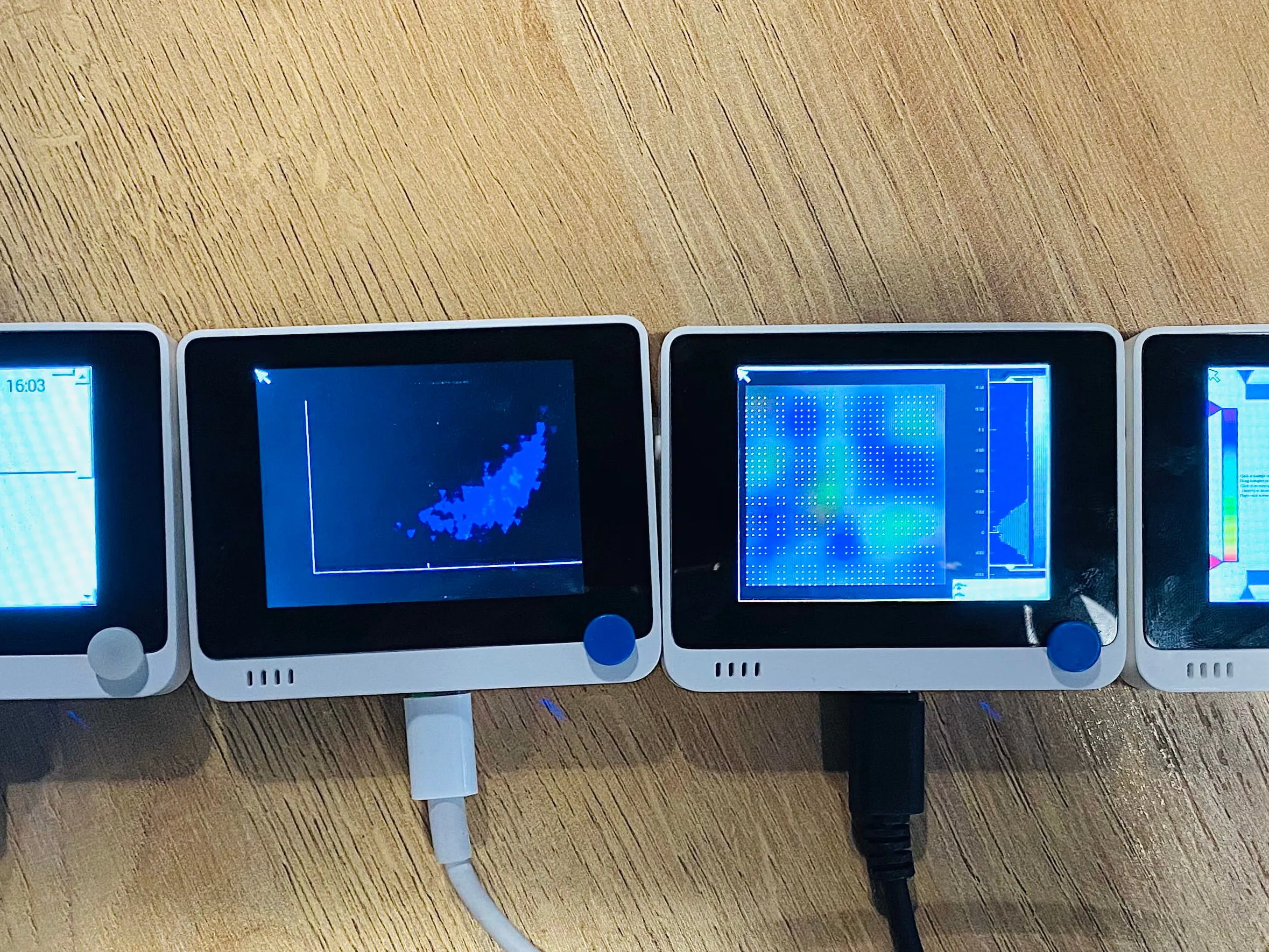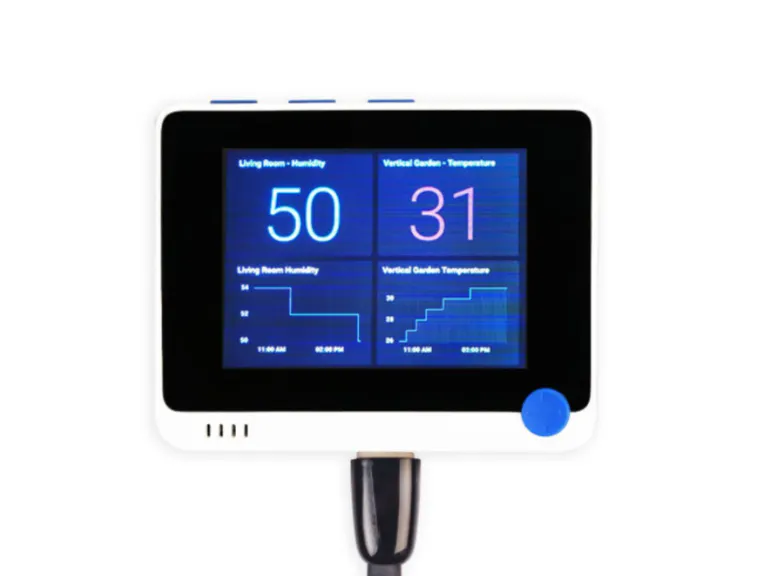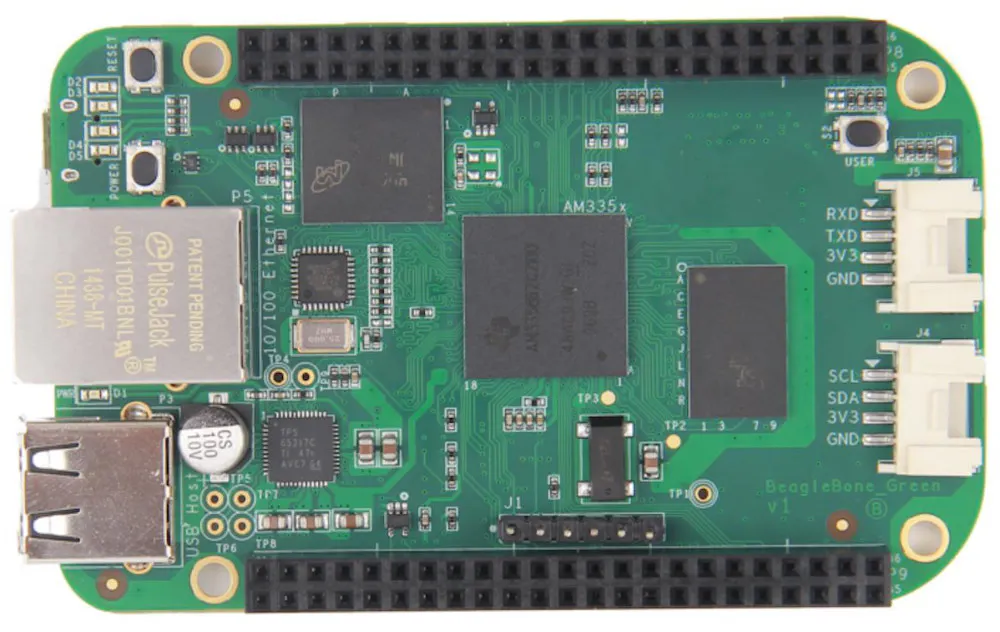# How to Use Wio Terminal as a USB HMI for BeagleBoard SBC

In this tutorial, I will demonstrate how to use Seeed Studio Wio Terminal as a USB HMI display for BeagleBone.

BeginnerProtip30 minutes1,495## Things used in this project

### Hardware componentsSeeed Studio Wio Terminal
×1BeagleBoard.org SeeedStudio BeagleBone Green
×1

## Code

### test.py

Python
PyQt test example
```# -*- coding: utf-8 -*-
"""
Demonstrates common image analysis tools.
Many of the features demonstrated here are already provided by the ImageView
widget, but here we present a lower-level approach that provides finer control
over the user interface.
"""
import pyqtgraph as pg
from pyqtgraph.Qt import QtCore, QtGui
import numpy as np

# Interpret image data as row-major instead of col-major
pg.setConfigOptions(imageAxisOrder='row-major')

pg.mkQApp()
win = pg.GraphicsLayoutWidget()
win.setWindowTitle('pyqtgraph example: Image Analysis')

# A plot area (ViewBox + axes) for displaying the image

# Item for displaying image data
img = pg.ImageItem()

# Custom ROI for selecting an image region
roi = pg.ROI([-8, 14], [6, 5])
roi.setZValue(10)  # make sure ROI is drawn above image

# Isocurve drawing
iso = pg.IsocurveItem(level=0.8, pen='g')
iso.setParentItem(img)
iso.setZValue(5)

# Contrast/color control
hist = pg.HistogramLUTItem()
hist.setImageItem(img)

# Draggable line for setting isocurve level
isoLine = pg.InfiniteLine(angle=0, movable=True, pen='g')
hist.vb.setMouseEnabled(y=False) # makes user interaction a little easier
isoLine.setValue(0.8)
isoLine.setZValue(1000) # bring iso line above contrast controls

# Another plot area for displaying ROI data
win.nextRow()
p2.setMaximumHeight(250)
win.resize(800, 800)
win.show()

# Generate image data
data = np.random.normal(size=(200, 100))
data[20:80, 20:80] += 2.
data = pg.gaussianFilter(data, (3, 3))
data += np.random.normal(size=(200, 100)) * 0.1
img.setImage(data)
hist.setLevels(data.min(), data.max())

# build isocurves from smoothed data
iso.setData(pg.gaussianFilter(data, (2, 2)))

# set position and scale of image
img.scale(0.2, 0.2)
img.translate(-50, 0)

# zoom to fit imageo
p1.autoRange()

# Callbacks for handling user interaction
def updatePlot():
global img, roi, data, p2
selected = roi.getArrayRegion(data, img)
p2.plot(selected.mean(axis=0), clear=True)

roi.sigRegionChanged.connect(updatePlot)
updatePlot()

def updateIsocurve():
global isoLine, iso
iso.setLevel(isoLine.value())

isoLine.sigDragged.connect(updateIsocurve)

def imageHoverEvent(event):
"""Show the position, pixel, and value under the mouse cursor.
"""
if event.isExit():
p1.setTitle("")
return
pos = event.pos()
i, j = pos.y(), pos.x()
i = int(np.clip(i, 0, data.shape - 1))
j = int(np.clip(j, 0, data.shape - 1))
val = data[i, j]
ppos = img.mapToParent(pos)
x, y = ppos.x(), ppos.y()
p1.setTitle("pos: (%0.1f, %0.1f)  pixel: (%d, %d)  value: %g" % (x, y, i, j, val))

# Monkey-patch the image to use our custom hover function.
# This is generally discouraged (you should subclass ImageItem instead),
# but it works for a very simple use like this.
img.hoverEvent = imageHoverEvent

## Start Qt event loop unless running in interactive mode or using pyside.
if __name__ == '__main__':
import sys
if (sys.flags.interactive != 1) or not hasattr(QtCore, 'PYQT_VERSION'):
QtGui.QApplication.instance().exec_()
```

## Credits

### Anson He

1 project • 0 followers